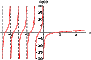I have forgotten

•http://facebook.com/
•https://www.google.com/accounts/o8/id
•https://me.yahoo.com

# Gamma

#### Log Gamma

Calculates the natural logarithm of the Gamma function with argument \e x.
 double log_gamma (double x)

#### Stirling

Stirling series approximation of the gamma function
 double stirling (double x)

#### Psi

Psi (digamma) function
 double psi (double x)#### Gamma Simple

A Sterling series approximation of the gamma function.
 double gamma_simple (double x)[inline]

#### Gamma

Returns gamma function of the argument.
 double gamma (double x, int *sign=NULL)

#### Gamma Lower Reg

The regularized lower incomplete Gamma integral
 double gammaLower_reg (double a, double x)

#### Gamma Upper Reg

The regularized upper incomplete Gamma integral
 double gammaUpper_reg (double a, double x)

#### Beta Reg

The regularized incomplete Beta integral
 double fraction_exp (double x, double a, double b, bool inverse=false) double betaLower_reg_pow (double x, double a, double b) double beta_reg (double x, double a, double b, bool upper = false)

#### Log Gamma Stirling

Natural Logarithm of Gamma function
 double log_gamma_stirling (double x, int* sign=NULL)

#### Log Gamma Simple

A Stirling series approximation of the gamma function.
 double logGamma_simple (double x)[inline]

#### Gamma Rcp

The reciprocal of the gamma function.
 double gamma_rcp (double x)

#### Log Gamma Frac

Evaluates ln(gamma(b) / gamma(a+b)).
 double logGammaFrac (double a, double b)

#### Log Gamma Sum

Returns the natural logarithm of the gamma function of the sum of two numbers.
 double logGammaSum (double a, double b)[inline]

#### Log Beta

Returns the natural logarithm of the complete beta function.
 double logBeta (double a, double b)

#### Beta Reg Inv

Inverse of the incomplete (regularized) beta integral.
 double df (double x, double a, double b, double logBeta)[inline] double fdx (double dx1, double x, double a, double b)[inline] double beta_reg_inv (double y, double a, double b, bool upper=false, double eps=1e-10) double beta_reg_inv_series (double y, double a, double b, double logBeta)[inline]

#### Gamma Upper Reg Inv

The inverse of the regularized upper incomplete Gamma integral
 double gammaUpper_reg_inv (double a, double y0)

#### Trigamma

Evaluates the Trigamma function.
 double trigamma (double x)

#### Beta

Evaluates the Beta function.
 double beta (double x, double y)